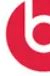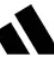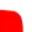Subtraction
Multiplication
Division
Mixed Review
100

0.95 + 5.09 =

6.04

100
5.68 - 4.23 =
1.45
100
8 x 6.5 =
52
100

13.8 / 3 =

4.6

100

For which 2 operations (addition, subtraction, multiplication, division) do you need to line up the decimal points?

200
11.27 + 9.4 =
20.67
200

Name this companyBeats

200
4.2 x 1.8 =
7.56
200

45.6 / 3

15.2

200

For which operations (addition, subtraction, multiplication, division) do you need to count the number of digits after the decimal points?

Multiplication

300

9.6 + 0.18 =

9.78

300

43.03 - 6.91 =

36.12

300

Name this company300

156 / 5 =

31.2

300

What number do you need to add to the dividend (after the decimal point) when there are no more numbers to bring down?

0

400

4.58 + 12.02 =

16.6

400

12 - 4.61 =

7.39

400

0.07 x 0.4

0.028

400

122 / 6.1

20

400

When multiplying by a decimal, which way do you move the decimal point?

To the left

500

8 + 2.45

10.45

500

62 - 48.6

13.4

500

.23 x 6.7

1.541

500

Name this company: